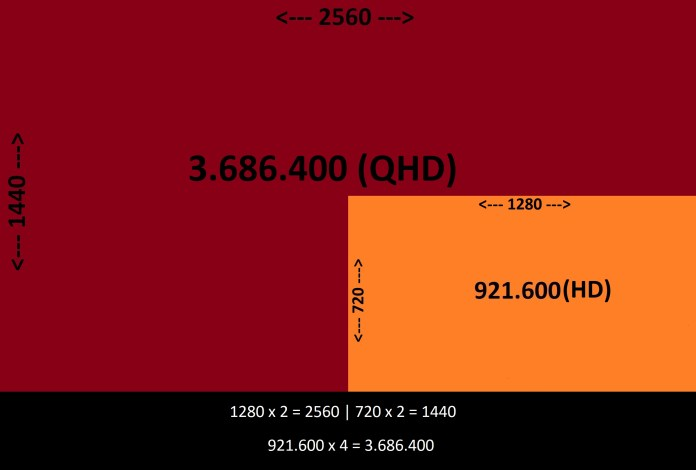# What are 1K - 2K - 4K - 8K Resolutions?What are 1K - 2K - 4K - 8K Resolutions?

The number of pixels displayed on a screen horizontally and vertically is called resolution. The more pixels there are on a screen, the more pixels the same image is divided into, and therefore the sharpness of the image will be increased. So the more pixels, the clearer and sharper the image.

The number of pixels written on screens, monitors and televisions also means this. For example, the phrase HD Ready (or 720p) written on old televisions would indicate that that television could display 1280 x 720 pixels. The Full HD screens that followed were devices with 1920 x 1080 pixel display.

So resolution concept is the number of pixels seen on a TV or monitor screen.

(DCI) Cinema resolution standards set by Digital Cinema Initiatives.

• DCI 1K = 1024(horizontal) x 540(vertical) = 552.960 pixels.
• DCI 2K = 2048 x 1080 = 2.211.840
• DCI 4K = 4096 x 2160 = 8.847.360
• DCI 8K = 8192 x 4320 = 35.389.440

DCI uses the phrase "K" horizontally / vertically for 1024 × 540 and its multiples. In other words, the number of pixels is 1024 horizontally, 540 times 2K in vertical and 2 times 4K.

2K has 1 times the resolution of 4K, or 4 times the total number of pixels. When the number of pixels in horizontal and vertical is doubled, the name "K" is doubled (Example: 2K / 2K), but the resolution is 4 times.

DCI 8K -> 4 times higher resolution than DCI 4K, 2 times higher than DCI 16K and 1 times higher than DCI 64K or more total pixel count.

What are the resolutions HD - Full HD - Quad HD - Ultra HD - Quad Ultra HD?

• HD = 1280(horizontal) x 720(vertical) = 921.600 pixels.
• Full HD (FHD) = 1920 x 1080 = 2.073.600
• Quad HD (QHD) = 2560 x 1440 = 3.686.400
• Ultra HD (UHD) = 3840 x 2160 = 8.294.400
• Quad Ultra HD (QUHD) = 7680 x 4320 = 33.177.600

In some places for example Wide Quad HD (W-QHD) or Ultra Wide Quad HD (UW-QHD). Here Wide (W) means wide meaning that the aspect ratio of the screen is 16: 9. It is not just any definition of resolution, as will be understood. The term Wide (W) is used for the aspect ratio 16: 9 and Ultra Wide (UW) for the 21: 9.

QHD is 2 times HD horizontally / vertically but has 4 times the resolution or total number of pixels. This is where the phrase "Quad" comes from there. Quad means quad, that is quad HD means 4 HD. QUHD means 4 UHDs.• 1280 x 3 = 3840 | 720 x 3 = 2160
• 3840 2160 x = 8.294.400
• 921.600 (HD) x 9 = 8.294.400 (UHD)
• 1920 1080 x = 2.073.600
• 1920 x 2 = 3840 | 1080 x 2 = 2160
• 2.073.600 (FHD) x 4 = 8.294.400 (UHD)